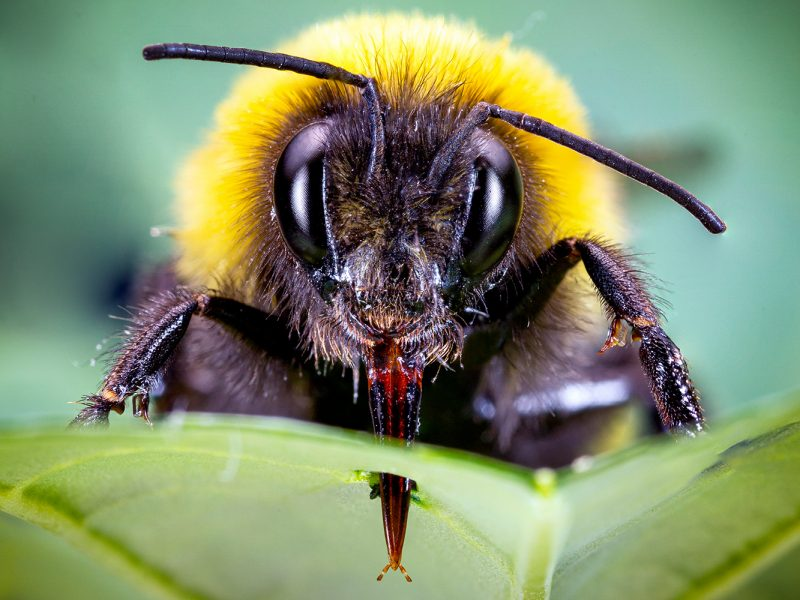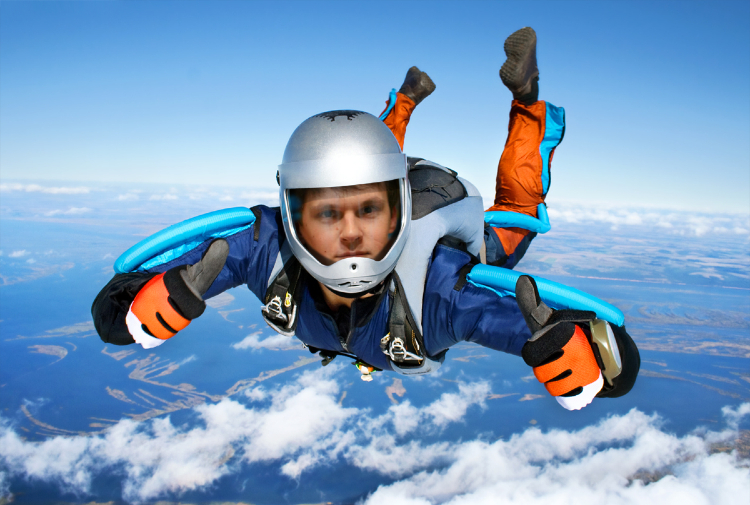Skip to main content

## Mahi Tuatahi: Kahoot!

Use your phones or laptops. Join as fast as you can!

https://create.kahoot.it/kahoots/my-kahoots/folder/2f5a1b63-53ba-4c44-9678-287ca08d461d

## What is Acceleration?

Acceleration is how quickly the velocity changes.

e.g. A supercar will accelerate to 50km/hr faster than a cyclist. That is to say, the supercar has a greater acceleration.

## Calculating Acceleration

\begin{aligned} acceleration &= \frac{\text{change in speed}}{\text{change in time}} \
a &= \frac{\Delta v}{\Delta t} \
\end{aligned}

• Velocity ($v$) has units meters per second ($ms^{-1}$)
• Time ($t$) has units seconds ($s$)
• Acceleration ($a$) has units meters per second per second ($m/s^{2}$ or $ms^{-2}$)

## Rearranging Equations

\begin{aligned} a &= \frac{\Delta v}{\Delta t} && \text{v is divided by t} \
a \times \Delta t &= \Delta v && \text{Undo the divide by multiplying} \
\Delta t &= \frac{\Delta v}{a} && \text{Undo the multiplication by dividing} \end{aligned}

\begin{aligned} v &= \frac{\Delta d}{\Delta t} && \text{d is divided by t} \
v \times \Delta t &= \Delta d && \text{Undo the divide by multiplying} \
\Delta t &= \frac{\Delta d}{v} && \text{Undo the multiplication by dividing} \end{aligned}

## Question 1

• A bee starts at rest and takes off from a flower and reaches a velocity of $0.75ms^{-1}$ in $0.5s$. Calculate it’s acceleration.## Question 1: Answer

1. Knowns: $\text{time (t)} = 0.5s$, $\Delta v = v_{f} - v_{i} = 0.75 - 0 = 0.75ms^{-1}$
2. Unknowns: $\text{acceleration (a)}$
3. Formula: $a = \frac{\Delta v}{\Delta t}$
4. Substitute: $a = \frac{0.75}{0.5}$
5. Solve: $v = 1.5\frac{m}{s^{2}} = 1.5ms^{-2}$

## Question 2

A Bugatti Veyron accelerates from rest at $27.77ms^{-2}$ for $2.6s$. How fast is it travelling at after 2.6 seconds?## Question 2: Answer

1. Knowns: $\text{time (t)} = 2.6s$, $\text{acceleration (a)} = 27.77ms^{-2}$
2. Unknowns: $\text{final velocity } v_{f}$
3. Formula: $a = \frac{\Delta v}{\Delta t} = \frac{v_{f} - v_{i}}{\Delta t}$
4. Substitute: $27.77 = \frac{v_{f} - 0}{2.6}$
5. Solve: $27.77 \times 2.6 = v_{f} = 72.2ms^{-1}$

## Question 3

A skydiver at rest jumps out of a plane. They accelerate at $9.8ms^{-2}$ until they reach a terminal velocity of $54ms^{-2}$. How long does it take them to reach this speed?## Question 3: Answer

1. Knowns: $\text{acceleration (a)} = 9.8ms^{-2}$, $\text{final velocity } (v_{f}) = 45ms^{-1}$
2. Unknowns: $\text{time (t)}$
3. Formula: $a = \frac{\Delta v}{\Delta t} = \frac{v_{f} - v_{i}}{\Delta t}$

\begin{aligned} 9.8 &= \frac{54 - 0}{t} \
9.8 \times t &= 54 \
t &= \frac{54}{9.8} = 5.51s \end{aligned}

## Question 4

A runner is approaching the finish line, moving at $5.55ms^{-1}$ but needs to sprint to pass the person just in front of them to get 1st place. They accelerate for $3s$ to reach $6.3ms^{-1}$. What is their acceleration?

## Question 4: Answer

1. Knowns: $v_{i} = 5.55ms^{-1}$, $v_{f} = 6.3ms^{-1}$, $\Delta t = 3s$
2. Unknowns: $\text{acceleration (a)}$
3. Formula: $a = \frac{\Delta v}{\Delta t} = \frac{v_{f} - v_{i}}{\Delta t}$

\begin{aligned} a &= \frac{6.3 - 5.55}{3} \
a &= \frac{0.75}{3} = 0.25ms^{-2} \end{aligned}

## Mahi Kāinga

• Due Monday, August 24th: Mahi Kāinga Booklet Q2, Q3, Q1
• Tutorials: Tuesday Lunchtime in A5
• Ask me for help with your mahi kāinga at any time!
• Do the work in your exercise book.